## How to count the number of characters, letters and numbers in cell?

When you type a list of data in a cell in Excel as shown as below screenshot, you want to count the total number of all characters, or only the number of the letters, or only the numbers in the cell. Now, I talk about the methods on this count in Excel.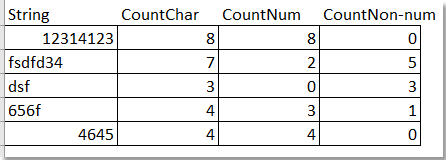If you want to count the total number of all characters, including numbers, letters and other marks in each cell, do it as follow:

1. Type this formula =LEN(A1) (the Cell A1 indicates the cell you want to count the total characters) into a blank cell, for example, the Cell B1, and click Enter button on the keyboard, and the total number of characters in Cell A1 has been counted. See screenshot: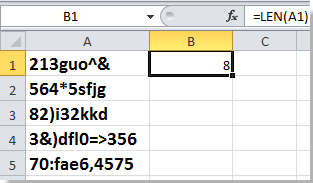2. Drag the fill handle to apply this formula to the range cells, and the number of characters in each cell of the list have been counted. See screenshot: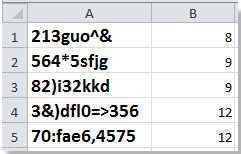If you only want to the amount of letters excluding numbers in each cell, you can do as follow:

Select a blank cell, for example, the Cell B1, type this formula

``=LEN(SUBSTITUTE(SUBSTITUTE(SUBSTITUTE(SUBSTITUTE(SUBSTITUTE(SUBSTITUTE(SUBSTITUTE(SUBSTITUTE(SUBSTITUTE(SUBSTITUTE(A1,0,""),1,""),2,""),3,""),4,""),5,""),6,""),7,""),8,""),9,""))``

(the Cell A1 indicates the cell you want to count amount of letters except numbers, you can change it as you need), then press Enter and drag the fill handle to fill the range you want to use this formula. See screenshot: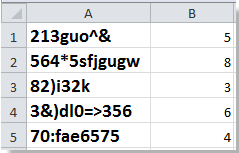Select a blank cell, for example, the Cell B1, type this formula =SUM(LEN(A1)-LEN(SUBSTITUTE(A1,{1,2,3,4,5,6,7,8,9,0},))) (the Cell A1 indicates the cell you want to count only amount of numbers, you can change it as you need), then press Enter and drag the fill handle to fill the range you want to use this formula. See screenshot: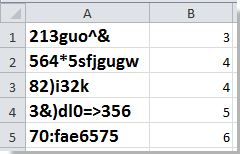With this function, you not only can know how many letters or numbers in the string of the cell, but also know the orders of the letters and numbers.

1. Hold ALT button and press F11 on the keyboard to open a Microsoft Visual Basic for Application window.

2. Click Insert > Module, and copy the VBA into the module.

VBA: Count amount of letters and numbers with function

``````Function AlphaNumeric(pInput As String) As String
'Updateby20140303
Dim xRegex As Object
Dim xMc As Object
Dim xM As Object
Dim xOut As String
Set xRegex = CreateObject("vbscript.regexp")
xRegex.Global = True
xRegex.ignorecase = True
xRegex.Pattern = "[^\w]"
AlphaNumeric = ""
If Not xRegex.test(pInput) Then
xRegex.Pattern = "(\d+|[a-z]+)"
Set xMc = xRegex.Execute(pInput)
For Each xM In xMc
xOut = xOut & (xM.Length & IIf(IsNumeric(xM), "N", "L"))
Next
AlphaNumeric = xOut
End If
End Function
``````

3. Save the code and close the window, and type this formula =AlphaNumeric(A1) ( the Cell A1 indicates the cell you want to count, you can change it as you need) into a blank cell, then press Enter and drag the fill handle to fill the range you want to use this formula. See screenshot: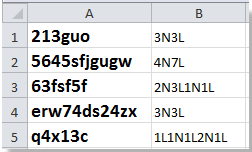Tip:

(1) The "L" indicates letter and "N" indicates number.

(2) This function does not work with cells containing special marks, such as !, @, #, \$, %, ^, &, etc.

If you want to count the number of a specific character in a string, for example, in the string “I want to count the number of a specific in a string”, I want to count the number of character “n”, how can you do?

In this case, I introduce Kutools for Excel’s COUNTCHAR function for you.

 with more than 300 handy functions, makes your jobs more easier. Free DownloadFull future 30 days free trial

After installing Kutools for Excel, please do as below:

1. Type the character you want to count in a cell, see screenshot: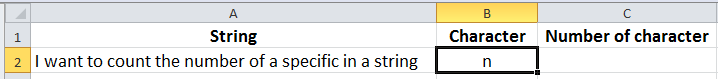2. Then select a blank cell to put the result and select a blank cell which will put the counting result, and click Kutools > Kutools Functions > Statistical & Math > COUNTCHAR. See screenshot: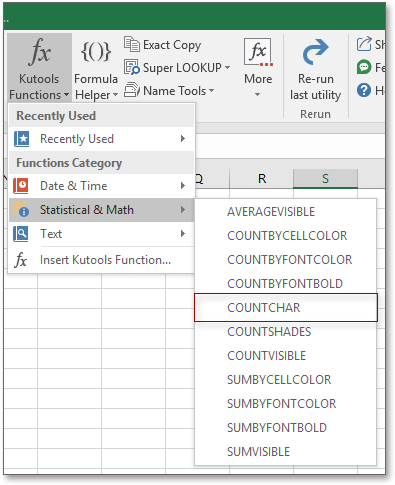3. Then in the popping Function Arguments dialog, select the string into Within_text box, and select the character cell into the Find_text box. Then you can see the counting result appears in the dialog.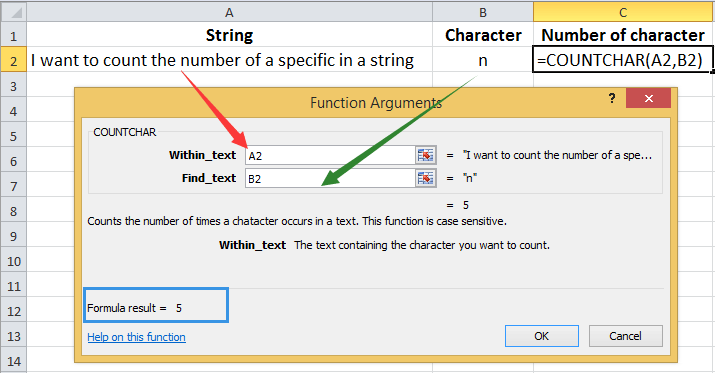4. Click OK, now the result is put in the cell you select.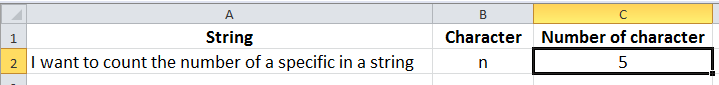In Kutools Functions, you can count data by background or font color, you can sum values by same background or font color, you can convert time to decimal hours/minute/seconds and so on.

#### Count times a word appears in an excel cell

If a word appears several times in a cell which needed to be count, usually, you may count them one by one. But if the word appears hundreds of times, the manually counting is troublesome. The Count times a word appears function in Kutools for Excel's Formula Helper group can quickly calculate the count of times a word appear in a cell. Free trial with full features in 30 days!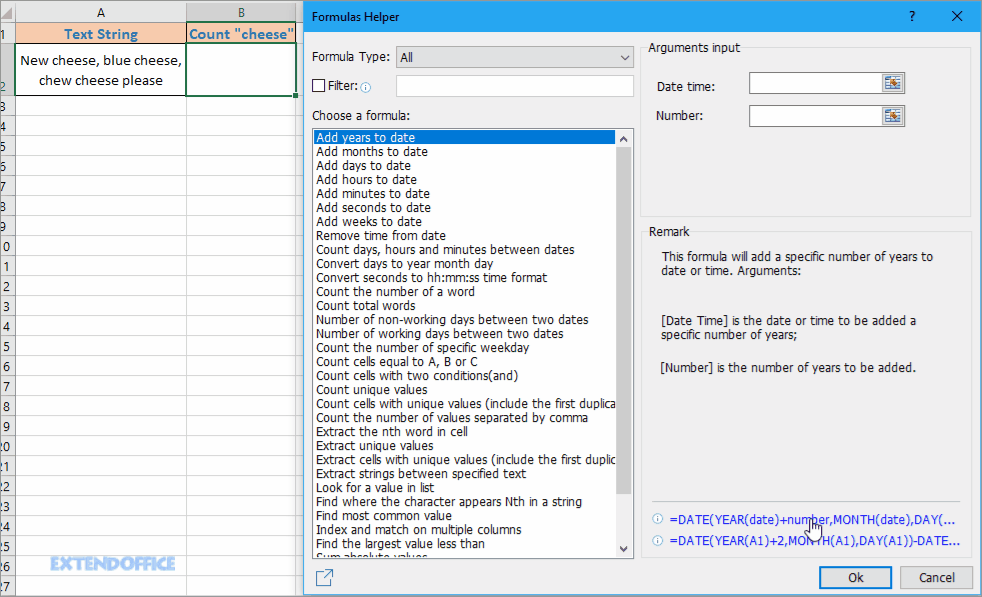Kutools for Excel: with more than 300 handy Excel add-ins, free to try with no limitation in 30 days.

### Best Office Productivity Tools

 Popular Features: Find, Highlight or Identify Duplicates   |  Delete Blank Rows   |  Combine Columns or Cells without Losing Data   |   Round without Formula ... Super Lookup: Multiple Criteria VLookup  |   Multiple Value VLookup  |   VLookup Across Multiple Sheets   |   Fuzzy Lookup .... Advanced Drop-down List: Quickly Create Drop Down List   |  Dependent Drop Down List   |  Multi-select Drop Down List .... Column Manager: Add a Specific Number of Columns  |  Move Columns  |  Toggle Visibility Status of Hidden Columns  |  Compare Ranges & Columns ... Featured Features: Grid Focus   |  Design View   |   Big Formula Bar   |  Workbook & Sheet Manager   |  Resource Library (Auto Text)   |  Date Picker   |  Combine Worksheets   |  Encrypt/Decrypt Cells   |  Send Emails by List   |  Super Filter   |   Special Filter (filter bold/italic/strikethrough...) ... Top 15 Toolsets:  12 Text Tools (Add Text, Remove Characters, ...)   |   50+ Chart Types (Gantt Chart, ...)   |   40+ Practical Formulas (Calculate age based on birthday, ...)   |   19 Insertion Tools (Insert QR Code, Insert Picture from Path, ...)   |   12 Conversion Tools (Numbers to Words, Currency Conversion, ...)   |   7 Merge & Split Tools (Advanced Combine Rows, Split Cells, ...)   |   ... and more

Supercharge Your Excel Skills with Kutools for Excel, and Experience Efficiency Like Never Before. Kutools for Excel Offers Over 300 Advanced Features to Boost Productivity and Save Time.  Click Here to Get The Feature You Need The Most...#### Office Tab Brings Tabbed interface to Office, and Make Your Work Much Easier

• Enable tabbed editing and reading in Word, Excel, PowerPoint, Publisher, Access, Visio and Project.
• Open and create multiple documents in new tabs of the same window, rather than in new windows.
• Increases your productivity by 50%, and reduces hundreds of mouse clicks for you every day!No ratings yet. Be the first to rate!
This comment was minimized by the moderator on the site
Excel của tôi là 2007
Đã nhiều lần làm công thức = LEN (SUBSTITUTE (SUBSTITUTE (SUBSTITUTE (SUBSTITUTE (SUBSTITUTE (SUBSTITUTE (SUBSTITUTE (SUBSTITUTE (SUBSTITUTE (SUBSTITUTE (SUBSTITUTE)
(A1,0,""),1,""),2,""),3,""),4,""),5,""),6,""),7,""),8,""),9,"")) mà ko thành công.
Mong Admin vui lòng trợ giúp!
Xin cám ơn!
This comment was minimized by the moderator on the site
Hi, maybe there is a line break in the formula which cause the error. I have modified the formula, please try again.
This comment was minimized by the moderator on the site
Thank you! Have bulleted statements in cells, have now been able to count them as individual items, brilliant!
This comment was minimized by the moderator on the site
Hi... how to set the formulas on Accuracy limits 98.1 to 103 - means exact 3 digits allow only.
This comment was minimized by the moderator on the site
How to enter this equation in excel
(4+y)/(2x+y)^2+12 whiles x=1 y=3 by naming x and y as 1 and 3 respectively
This comment was minimized by the moderator on the site
Can solve it for me.. Iwant count of this cell :-
1+1.5+2+1.5+2.5+1+3+3.5

1=?, 1.5=?, 2=?, 2.5=?, 3=?, 3.5=?... using LEN but ans.wrong (1=4, 1.5=2, 2=2, 2.5=1, 3=2, 3.5=1)

This comment was minimized by the moderator on the site
1+2+3+4+5+6 I need help with this one. How can I ACTUALLY figure this out...?
This comment was minimized by the moderator on the site
It was a little Confusing but I knew this will be a good help for me because I just knew typing like coding this was such a good idea in order thx very much.
This comment was minimized by the moderator on the site
I thank you for how I understand what that meant?
This comment was minimized by the moderator on the site
Hello, How can I check for password validation that is stored as excel cell data using vba script and display message for all the wrong entries showing the location of error?
This comment was minimized by the moderator on the site
Hi excel experts,
Count only numbers within a cell, excluding letters & other characters
So, let's say we have 1, 4, 5, 6. I'd like A2 to show "4".

Formula used =SUM(LEN(X4)-LEN(SUBSTITUTE(X4,{1,2,3,4,5,6,7,8,9,0},))) so, Will be single digit OK. but two digit wrong formula.

But Double digit number how to count...?

so any double digit number, e.g., 10
This comment was minimized by the moderator on the site
Hi，vivek kachhadiya, you can use =EXTRACTNUMBERS(cell) to extract all numbers from string to a cell, then use =LEN(cell) to count the number of numbers.
See screenshot:
There are no comments posted here yet